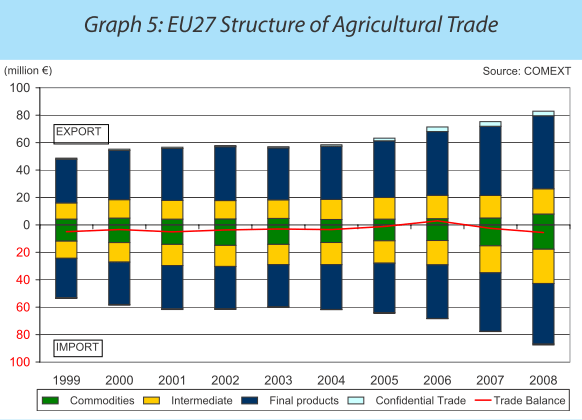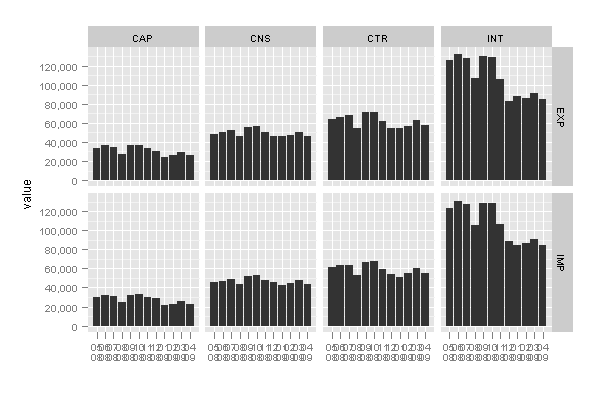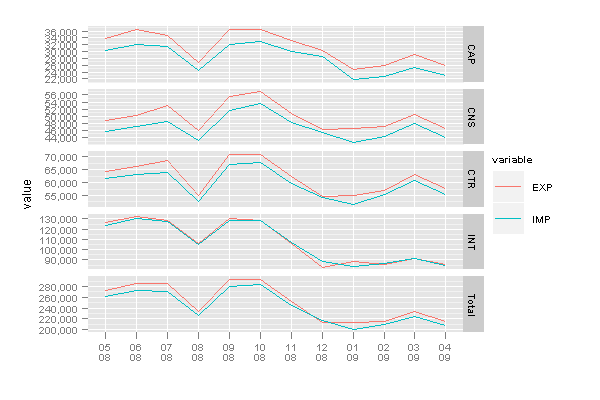# ggplot2: Back-to-back Bar Charts

September 23, 2009
By

Want to share your content on R-bloggers? click here if you have a blog, or here if you don't.

On the ggplot2 mailing-list the following question was asked:

How to create a back-to-back bar chart with ggplot2?

For anyone who don’t know what I am talking about, have a look on a recent paper from the EU. I’d like to create plots like the graphs 5,6,18 in the paper.

An example graph from the above report is below:Let’s create the same graph in ggplot2.

I was not able to find the exact dataset used to plot the graph above, and used instead the Eurostat “EU27 trade by BEC product group since 1999″ dataset which has a very similar data structure.

Access the subset used in this post in here.

 `> library(ggplot2)`
 ```> trade <- read.csv("trade.csv", header = TRUE, stringsAsFactors = FALSE)```

 ```> balance <- ddply(trade, .(Time), summarise, balance = sum(EXP - IMP))```

Convert data from wide format to long format for plotting.

 `> trade.m <- melt(trade, id.vars = c("BEC", "Time"))`
 ```> ggplot(trade.m, aes(Time)) + geom_bar(subset = .(variable == "EXP"), aes(y = value, fill = BEC), stat = "identity") + geom_bar(subset = .(variable == "IMP"), aes(y = -value, fill = BEC), stat = "identity") + xlab("") + scale_y_continuous("Export - Import", formatter = "comma")``````> labels <- gsub("20([0-9]{2})M([0-9]{2})", "\2n\1", trade.m\$Time) > last_plot() + geom_line(data = balance, aes(Time, balance, group = 1), size = 1) + geom_hline(yintercept = 0, colour = "grey90") + scale_x_discrete(labels = labels)```The legend explanation (too large to fit on the plot):

• CAP Capital goods
• CNS Consumption goods
• CTR Consumption goods plus motor spirit and passenger motor cars
• INT Intermediate goods

There shouldn’t be any negative numbers on the y-axis, we need a custom formatter to convert these to positive.

 ```> commapos <- function(x, ...) { format(abs(x), big.mark = ",", trim = TRUE, scientific = FALSE, ...) }```
 `> last_plot() + scale_y_continuous(formatter = "commapos")`I have to agree with some of the posters on the mailing list, that this type of chart is not very easy to follow – it is impossible to compare individual values. Another useful way of showing information would be to use facets:

 ```> ggplot(trade.m, aes(Time)) + geom_bar(aes(y = value), stat = "identity") + facet_grid(variable ~ BEC) + scale_x_discrete("", labels = labels) + scale_y_continuous(formatter = "comma")```Or combine export and import values on one plot showing the comparative trend between the different goods categories.

 ```> ggplot(trade.m, aes(Time, colour = variable, group = variable)) + geom_line(aes(y = value)) + facet_grid(BEC ~ .) + scale_x_discrete("", labels = labels) + scale_y_continuous(formatter = "comma")```The same graph with varying scales across panels.

 `> last_plot() + facet_grid(BEC ~ ., scales = "free_y")`Update: As suggested in the comments it would be good to have total values added to the last plot.

In order to do so the totals are first calculated and then appended to the original dataframe.

 ```> total <- ddply(trade.m, .(Time, variable), summarise, value = sum(value), BEC = "Total") > total <- rbind(trade.m, total)```

Plotting the new dataframe is just a question of changing the source of the last plot.

 `> last_plot() %+% total`R-bloggers.com offers daily e-mail updates about R news and tutorials about learning R and many other topics. Click here if you're looking to post or find an R/data-science job.
Want to share your content on R-bloggers? click here if you have a blog, or here if you don't.

Tags: , , ,